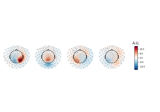# mne.decoding.SSD¶

class mne.decoding.SSD(info, filt_params_signal, filt_params_noise, reg=None, n_components=None, picks=None, sort_by_spectral_ratio=True, return_filtered=False, n_fft=None, cov_method_params=None, rank=None)[source]

M/EEG signal decomposition using the Spatio-Spectral Decomposition (SSD).

SSD seeks to maximize the power at a frequency band of interest while simultaneously minimizing it at the flanking (surrounding) frequency bins (considered noise). It extremizes the covariance matrices associated with signal and noise 1.

SSD can either be used as a dimensionality reduction method or a ‘denoised’ low rank factorization method 2.

Parameters
infoinstance of `mne.Info`

The info object containing the channel and sampling information. It must match the input data.

filt_params_signal`dict`

Filtering for the frequencies of interest.

filt_params_noise`dict`

Filtering for the frequencies of non-interest.

reg`float` | `str` | `None` (default)

Which covariance estimator to use. If not None (same as ‘empirical’), allow regularization for covariance estimation. If float, shrinkage is used (0 <= shrinkage <= 1). For str options, reg will be passed to method to `mne.compute_covariance()`.

n_components

The number of components to extract from the signal. If n_components is None, no dimensionality reduction is applied.

picks

The indices of good channels.

sort_by_spectral_ratiobool (default `False`)

If set to True, the components are sorted accordingly to the spectral ratio. See Eq. (24) in 1.

return_filteredbool (default `True`)

If return_filtered is True, data is bandpassed and projected onto the SSD components.

n_fft`int` (default `None`)

If sort_by_spectral_ratio is set to True, then the SSD sources will be sorted accordingly to their spectral ratio which is calculated based on `mne.time_frequency.psd_array_welch()` function. The n_fft parameter set the length of FFT used. See `mne.time_frequency.psd_array_welch()` for more information.

cov_method_params

As in `mne.decoding.SPoC` The default is None.

rank`None` | `dict` | ‘info’ | ‘full’

As in `mne.decoding.SPoC` This controls the rank computation that can be read from the measurement info or estimated from the data. See Notes of `mne.compute_rank()` for details. We recommend to use ‘full’ when working with epoched data.

References

1(1,2,3)

Vadim V Nikulin, Guido Nolte, and Gabriel Curio. A novel method for reliable and fast extraction of neuronal EEG/MEG oscillations on the basis of spatio-spectral decomposition. NeuroImage, 55(4):1528–1535, 2011. doi:10.1016/j.neuroimage.2011.01.057.

2(1,2)

Stefan Haufe, Sven Dähne, and Vadim V Nikulin. Dimensionality reduction for the analysis of brain oscillations. NeuroImage, 101:583–597, 2014. doi:https://doi.org/10.1016/j.neuroimage.2014.06.073.

Attributes
filters_`array`, shape (n_channels, n_components)

The spatial filters to be multiplied with the signal.

patterns_`array`, shape (n_components, n_channels)

The patterns for reconstructing the signal from the filtered data.

Methods

 `__hash__`(/) Return hash(self). Remove selected components from the signal. `fit`(X[, y]) Estimate the SSD decomposition on raw or epoched data. `fit_transform`(X[, y]) Fit to data, then transform it. `get_params`([deep]) Get parameters for this estimator. `get_spectral_ratio`(ssd_sources) Get the spectal signal-to-noise ratio for each spatial filter. Not implemented yet. `set_params`(**params) Set the parameters of this estimator. Estimate epochs sources given the SSD filters.
apply(X)[source]

Remove selected components from the signal.

This procedure will reconstruct M/EEG signals from which the dynamics described by the excluded components is subtracted (denoised by low-rank factorization). See 2 for more information.

Note

Unlike in other classes with an apply method, only NumPy arrays are supported (not instances of MNE objects).

Parameters
Xarray, shape ([n_epochs, ]n_channels, n_times)

The input data from which to estimate the SSD. Either 2D array obtained from continuous data or 3D array obtained from epoched data.

Returns
Xarray, shape ([n_epochs, ]n_channels, n_times)

The processed data.

fit(X, y=None)[source]

Estimate the SSD decomposition on raw or epoched data.

Parameters
Xarray, shape ([n_epochs, ]n_channels, n_times)

The input data from which to estimate the SSD. Either 2D array obtained from continuous data or 3D array obtained from epoched data.

y`None` | `array`, shape (n_samples,)

Used for scikit-learn compatibility.

Returns
selfinstance of `SSD`

Returns the modified instance.

Examples using `fit`:fit_transform(X, y=None, **fit_params)[source]

Fit to data, then transform it.

Fits transformer to X and y with optional parameters fit_params and returns a transformed version of X.

Parameters
X`array`, shape (n_samples, n_features)

Training set.

y`array`, shape (n_samples,)

Target values.

**fit_params`dict`

Additional fitting parameters passed to `self.fit`.

Returns
X_new`array`, shape (n_samples, n_features_new)

Transformed array.

get_params(deep=True)[source]

Get parameters for this estimator.

Parameters
deepbool, optional

If True, will return the parameters for this estimator and contained subobjects that are estimators.

Returns
params`dict`

Parameter names mapped to their values.

get_spectral_ratio(ssd_sources)[source]

Get the spectal signal-to-noise ratio for each spatial filter.

Spectral ratio measure for best n_components selection See 1, Eq. (24).

Parameters
ssd_sources`array`

Data projected to SSD space.

Returns
spec_ratio`array`, shape (n_channels)

Array with the sprectal ratio value for each component.

sorter_spec`array`, shape (n_channels)

Array of indices for sorting spec_ratio.

References

Examples using `get_spectral_ratio`:inverse_transform()[source]

Not implemented yet.

set_params(**params)[source]

Set the parameters of this estimator.

The method works on simple estimators as well as on nested objects (such as pipelines). The latter have parameters of the form `<component>__<parameter>` so that it’s possible to update each component of a nested object.

Parameters
**params`dict`

Parameters.

Returns
instinstance

The object.

transform(X)[source]

Estimate epochs sources given the SSD filters.

Parameters
Xarray, shape ([n_epochs, ]n_channels, n_times)

The input data from which to estimate the SSD. Either 2D array obtained from continuous data or 3D array obtained from epoched data.

Returns
X_ssdarray, shape ([n_epochs, ]n_components, n_times)

The processed data.

Examples using `transform`:## Examples using `mne.decoding.SSD`¶